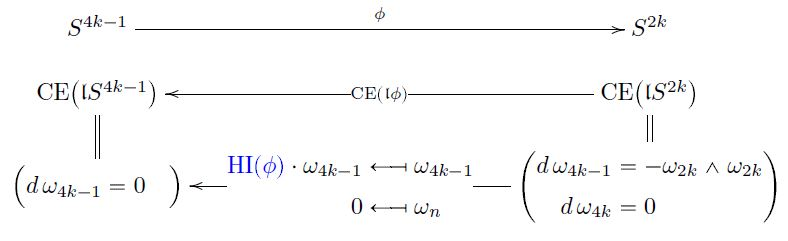Contents

# Contents

## Definition

### In ordinary cohomology

For $n \in \mathbb{N}$ with $n \gt 1$, consider continuous functions between spheres of the form

(1)$f \;\colon\; S^{2n-1} \longrightarrow S^n \,.$

The homotopy cofiber of $f$ (the attaching space induced by $f$)

$C_f \;\coloneqq\; S^n \underset{S^{2n-1}}{\cup} D^{2n}$
$H^k(C_f, \mathbb{Z}) \simeq \left\{ \array{ \mathbb{Z} & for\; k = n, 2n; \\ 0 & otherwise } \right. \,.$

Hence for $\alpha_n, \beta_{2n}$ generators of the cohomology groups in degree $n$ and $2n$ (unique up to choice of sign), respectively, there exists an integer $HI(f)$ which expresses the cup product square of $\alpha_n$ as a multiple of $\beta_{2n}$:

(2)$\alpha_n \cup \alpha_n \;=\; HI(f) \cdot \beta_{2n} \,.$

This integer $HI(f) \in \mathbb{Z}$ is called the Hopf invariant of $f$ (e.g. Mosher-Tangora 86, p. 33).

This depends on the choices made only up to sign. In particular it has a well-defined mod-2 reduction image $[HI(f)] \in \mathbb{F}_2 = \mathbb{Z}/2\mathbb{Z}$ (in Z/2), and as such it is the Steenrod square

$[HI(f)] \cdot (-) \;\colon\; \mathbb{F}_2 \;\simeq\; H^n \big( C_f; \, \mathbb{F}_2 \big) \stackrel{Sq^n}{\longrightarrow} H^{2n} \big( C_f; \, \mathbb{F}_2 \big) \;\simeq\; \mathbb{F}_2 \,.$

### In generalized cohomology

Here is a more abstract picture of the Hopf invariant in abstract homotopy theory (following SS21):

Let $E$ be a multiplicative cohomology theory, assumed to vanish in degree $2n - 1$

(3)$\widetilde E \big( S^{2n-1} \big) \,\simeq\, \pi_{2n-1}(E) \,\simeq\, 0 \,.$

We write $E_n \;\coloneqq\; \Omega^\infty \Sigma^n E$ for its classifying spaces, hence for the component spaces of its representing spectrum.

(For the case of ordinary cohomology $E = H \mathbb{Z}$ we have $E_n \,\simeq\, K(\mathbb{Z},n)$ an Eilenberg-MacLane space.)

Now let

$S^{2n-1} \overset{f}{\longrightarrow} S^n$

be a map (1). Then its $E$-Hopf invariant “is” the following homotopy pasting diagram of pointed homotopy types (see also at e-invariant is Todd class of cobounding (U,fr)-manifold):

• the top square is defined to be a homotopy pushout, exhibiting the attaching space $C_f$;

• the total rectangle is defined to be a homotopy pushout, exhibiting the suspension of $S^{2n-1}$ to $S^{2n}$;

• the bottom square is hence a homotopy pushout by the pasting law;

• by assumption (3) the restriction of $\Sigma^{2n} 1$ to $S^{2n-1}$ trivializes, exhibited by a choice of homotopy filling the full top part of the diagram; and by the universal property of the top homotopy-pushout this corresponds equivalently to the dashed morphism $c$;

• the restriction of the cup square cohomology operation on $c$ to $S^n$ trivializes: $c$ factors through the $n$ component space of the connective cover $E\langle 0\rangle$, whence its cup square (by the discussion at connective cover – For ring spectra) factors through the $2n$-connective $2n$th component space of the same connective cover (as in a standard argument in complex oriented cohomology theory, e.g. Lurie, Lec. 4. Exmpl. 8, or see Conner-Floyd 66, Part I, Cor. 7.2):

• This yields the homotopy filling the full bottom part of the diagram above; and by the universal property of the bottom homotopy-pushout this corresponds equivalently to a dashed morphism $S^{2n} \to E_{2n}$, labeled by some class

$\kappa \,\in\, \pi_0(E) \,\simeq\, \widetilde E(S^0) \overset{\Sigma^{2n}}{\longrightarrow} \widetilde E(S^{2n}) \, = \, \big[ S^{2n} \longrightarrow E_{2n} \big] \,;$
• finally, by the homotopy-pushout property of the total rectangle, this class $\kappa$ also labels the total homotopy filling the full diagram.

We see that:

• for $E = H \mathbb{Z}$ being ordinary cohomology, $\kappa \,\in\, \mathbb{Z}$ is the traditional Hopf invariant as discussed above;

• for $E = KU$ being complex topological K-theory, $\kappa \,\in\, \mathbb{Z}$ is the Hopf invariant in K-theory as used in Adams-Atiyah 66.

In the case that the map $f$ is one the classical Hopf fibrations, the attaching space above is a projective space (by the discussion at cell structure of projective spaces) and the choice of homotopy $c$ is the choice of an orientation in $E$-cohomology theory to second stage. Specifically:

Moreover, the de-composition of this pasting diagram exhibits the homotopy Whitehead integral/functional cup product-formula for the Hopf invariant:

## Properties

### Generic values

For $n$ odd, the Hopf invariant necessarily vanishes. For $n$ even however, then there is a homomorphism

$\pi_{2n-1}(S^n) \longrightarrow \mathbb{Z}$

whose image contains at least the even integers.

### Hopf invariant one

A famous open question in the 1950s was which maps $f$ (1) have Hopf invariant one, namely $[HI(f)] = 1$ (2).

The Hopf invariant one theorem (Adams60) states that the only maps of Hopf invariant one, $[HI(f)] = 1$, are the Hopf constructions on the four real normed division algebras:

### Via Sullivan models

###### Proposition

By standard results in rational homotopy theory, every continuous function

$S^{4k-1} \overset{f}{\longrightarrow} S^{2k}$

corresponds to a unique dgc-algebra homomorphism

$CE \big( \mathfrak{l}S^{4k-1} \big) \overset{ CE(\mathfrak{l}f) }{\longleftarrow} CE \big( \mathfrak{l}S^{2k} \big)$

between Sullivan models of n-spheres.

The unique free coefficient of this homomorphism $CE(\mathfrak{l}f)$ is the Hopf invariant $HI(f)$ of $f$: